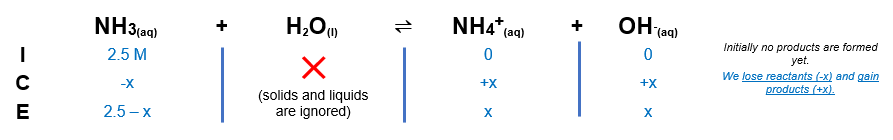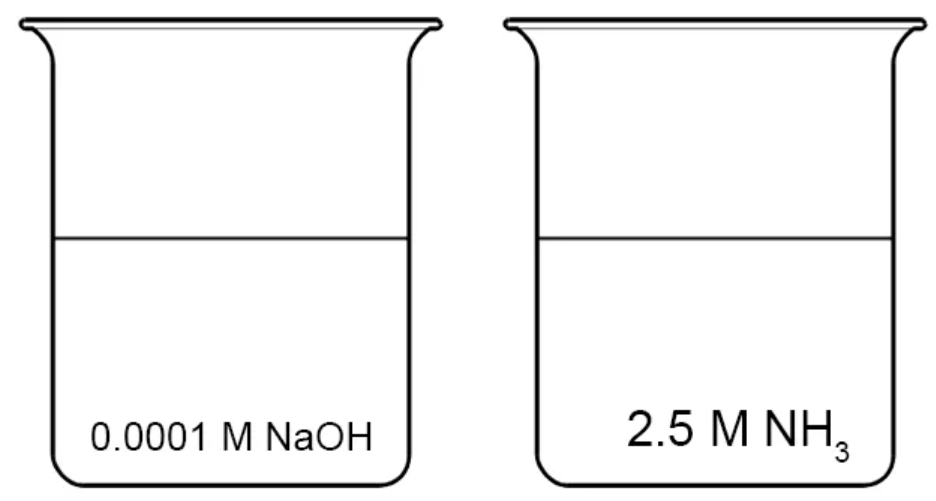# Problem: How does the pH of the solution on the right compare with that of the solution on the left? The Kb of NH3 is 1.8 x 10-5.A.) Both of these solutions are equally acidic.B.) The solution on the right is neutral relative to the solution on the left.C.) The solution on the right is basic relative to the solution on the left.D.) Both of these solutions are equally basic.E.) The solution on the right is acidic relative to the solution on the left

###### FREE Expert Solution

We need to compare the OHconcentrations.

↑ [OH-] → more basic → less acidic

Calculate the [OH-] for 2.5 M NH3

Writing the Kb expression for the given reaction:

NH3(aq) + H2O(l)  ⇌  NH4+(aq) + OH-(aq)
(reactants)              (products)91% (346 ratings)###### Problem Details

How does the pH of the solution on the right compare with that of the solution on the left? The Kb of NH3 is 1.8 x 10-5.A.) Both of these solutions are equally acidic.
B.) The solution on the right is neutral relative to the solution on the left.
C.) The solution on the right is basic relative to the solution on the left.
D.) Both of these solutions are equally basic.
E.) The solution on the right is acidic relative to the solution on the left